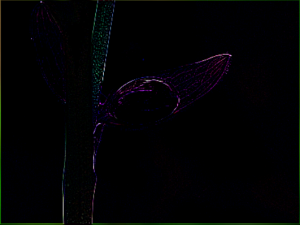GeeksforGeeks App
Open AppBrowser
Continue

# Python PIL | Kernel() method

PIL is the Python Imaging Library which provides the python interpreter with image editing capabilities. The ImageFilter module contains definitions for a pre-defined set of filters, which can be used with the `Image.filter() `method.

`PIL.ImageFilter.Kernel()` Create a convolution kernel. The current version only supports 3×3 and 5×5 integer and floating point kernels.

Syntax: PIL.ImageFilter.Kernel(size, kernel, scale=None, offset=0)

Parameters:
size – Kernel size, given as (width, height). In the current version, this must be (3, 3) or (5, 5).
kernel – A sequence containing kernel weights.
scale – Scale factor. If given, the result for each pixel is divided by this value. the default is the sum of the kernel weights.
offset – Offset. If given, this value is added to the result, after it has been divided by the scale factor.

Returns type: An image.

Image Used:`  ` ` ` `# Importing Image and ImageFilter module from PIL package ``from` `PIL ``import` `Image, ImageFilter `` ` `# creating a image object ``im1 ``=` `Image.``open``(r``"C:\Users\System-Pc\Desktop\leave.JPG"``) `` ` `# applying the Kernel filter``im2 ``=` `im1.``filter``(ImageFilter.Kernel((``3``, ``3``),``      ``(``-``1``, ``-``1``, ``-``1``, ``-``1``, ``9``, ``-``1``, ``-``1``, ``-``1``, ``-``1``), ``1``, ``0``))`` ` `im2 ``=` `im2.show()                 `

Output:Another example: Here change kernel value to obtain output, we can change other parameters as well.

 `# Importing Image and ImageFilter module from PIL package ``from` `PIL ``import` `Image, ImageFilter `` ` `# Importing Image and ImageFilter module from PIL package ``from` `PIL ``import` `Image, ImageFilter `` ` `# creating a image object ``im1 ``=` `Image.``open``(r``"C:\Users\System-Pc\Desktop\leave.JPG"``) `` ` `# applying the Kernel filter``im2 ``=` `im1.``filter``(ImageFilter.Kernel((``3``, ``3``),``          ``(``-``1``, ``-``1``, ``-``1``, ``-``1``, ``11``, ``-``2``, ``-``2``, ``-``2``, ``-``2``), ``1``, ``0``))`` ` `im2 ``=` `im2.show()                 `

Output:My Personal Notes arrow_drop_up